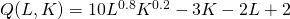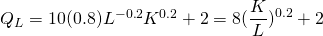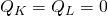# Solution assignment 06 Partial differentiation

### Assignment 6

Calculate the first partial derivatives of the following function.### Solution

In economics this function is known as a production function. It is quite easy to calculate the first partial derivatives.The second partial derivatives are less relevant. In all cases the first derivatives are necessary to find the extremes, by solving the equations. Calculating the second and mixed partial derivatives is a lot of work, so we do not calculate them here.